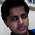### Need for Needles

Source: Quantnet Forums

Problem:
You are given a stick of length 1 and a supply of n identical needles of length h. Drop the n needles at random on the stick, subject to the following "needle discipline": needles should fit entirely within the stick (they cannot stick out). What is the probability that no two needles overlap? Let us assume that the stick is one-dimensional, so that the needles can only lie along the length of the stick.

Update (26-05-2011)
Solution:
Posted by Siddhant Agarwal (EE IITB 2011 Alumnus), Ameya Ranade (CSE IITB 2009 Alumnus) and me in comments!

1.ans = 0 if (1-nh) <=0
= [(1-nh)/(1-h)]^n otherwise

simple n dimentional integration. However cant think of an elegant solution

2.We have n! permutations for arrangment of sticks properly. Lets integrate from length i'th stick leaves for rest n-i sticks to be put. (Ai)
Which gives us :
Prob. = (l-h)^n * IGR[(n-1)h to l-h] of (IGR[(n-2)h to Ai] of (IGR.... (IGR[h to An-2] dAn-1 dAn-2.. dA1.
Substituting Bi = Ai - (n-i)h,
It solves to 1/n! * ((l-nh)/(l-h))^n
Multiplying by n! for total number of ways to arrange sticks,
we get ((l-nh)/(l-h))^n

3.Dropping the needles so that they all fall within the stick is equivalent to randomly picking n numbers (the left endpoints) each uniformly and independently drawn from [0,1-h]. Under this interpretation, the volume of the sample space is (1-h)^n. The volume of the region where the first needle is to the left of the second is to the left of the third ... is to the left of the nth is given by n-dimensional integral as in

Equation Image
Solving this gives [(1-nh)/(1-h)]^n

4.I solved this problem using some intuition, not sure if it is entirely rigorous...
Consider x_1,x_2,...,x_n+1
x_1 is the distance from the left end of the stick to the left end of the first needle
x_n+1 is the distance from the right end of the last needle to the right end of the stick
x_i is the displacement (+ve or -ve) from the right end of the i-th needle to the left end of the (i-1)th needle (i=2,3,4,...,n)
Now, for any arrangement,
x_1 + x_2 +...+ x_n+1 = 1-nh
0<= x_1,x_n+1 <=1-h
For the sample space: -h <= x_2,x_3,...,x_n <= 1-2h
For the valid cases: 0 <= x_2,x_3,...,x_n <= 1-nh
Define y_i = x_i + h

Sample space is the solution space for:
x_1 + y_2 + y_3 +...+ y_n + x_n+1 = 1-h
0 <= x_1,y_2,y_3,...,y_n,x_n+1 <= 1-h

Valid cases is the solution space for:
x_1 + x_2 +... + x_n+1 = 1-nh
0 <= x_1,x_2,...,x_n+1 <= 1-nh

Suppose we have a k dimensional hyper-space. Then the area of the (k-1) dimension hyper-plane formed by using k distinct unit vectors, each scaled by a factor 'a' is equal to c_k*a^(k-1), where c_k is some constant for a k dimensions [This is based on intuition. It would be great if someone could provide a formal proof for the same].

The solution space for the sample space and the valid space is a n-dimensional hyper-plane on a (n+1) dimension hyper-space. For the sample space, the unit vectors are scaled by a factor of (1-h) while for the valid space, the scaling factor is (1-nh).

Using this, the probability comes out to be [(1-nh)/(1-h)]^n

5.Minor correction:
x_i is the displacement (+ve or -ve) from the right end of the i-th needle to the left end of the (i+1)th needle, NOT (i-1)th NEEDLE (i=2,3,4,...,n)